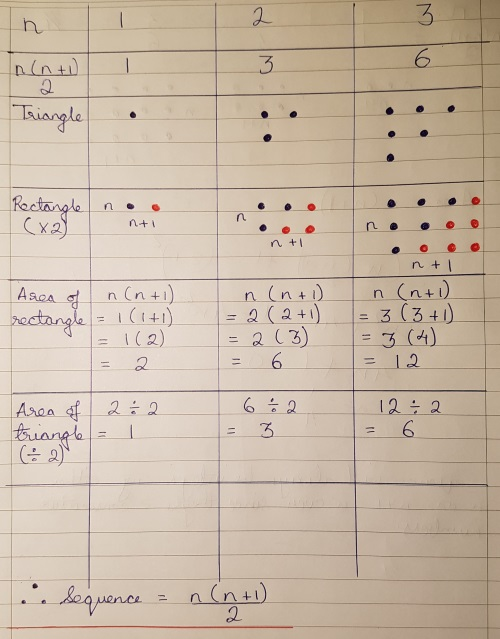#### You may also like### Proof Sorter - Quadratic Equation

This is an interactivity in which you have to sort the steps in the completion of the square into the correct order to prove the formula for the solutions of quadratic equations.### Proof Sorter - Geometric Sequence

Can you correctly order the steps in the proof of the formula for the sum of the first n terms in a geometric sequence?### Proof Sorter - the Square Root of 2 Is Irrational

Try this interactivity to familiarise yourself with the proof that the square root of 2 is irrational. Sort the steps of the proof into the correct order.

# Iff

##### Age 14 to 18Challenge Level
We had some great solutions to this problem, including both algebraic and geometric approaches.
Navjot from Sherborne Qatar sent us a diagram showing why the triangular numbers can be expressed using the formula $T_n=\frac12 n(n+1)$:Rishika from Nonsuch High and Navjot both showed how to calculate the quadratic sequence by using differences. Here is Rishika's method:

Triangular number sequence: 1   3   6   10”¦

I noticed that this was a quadratic sequence, with the first differences
being 1,2,3,4.. and the second common difference being 1.

1    3    6    10    a+b+c
2   3     4        3a+b
1    1            2a

I then used the above expressions to solve and obtain the quadratic sequence rule:
$2a=1 \Rightarrow a=0.5$

$3(0.5) +b = 2$
$1.5 +b = 2$
$b = 0.5$

$a+b+c = 1$
$0.5+0.5+c = 1$
$c = 0$

Therefore, the $k^{th}$ term of the triangular number sequence is $0.5k^2 +0.5k$

Rishika, Navjot, Emily from South Hunsley and Samuel from West Bridgnorth all showed that if you multiply this by 8 and add 1, you always get a square number. Here's how Samuel worked it out:

$T_n = \frac{n^2+n}2$
$8T_n = 4n^2+4n$
$8T_n + 1 = 4n^2+4n+1$
This factorises to give $(2n+1)^2$

Eleanor from North Yorkshire gave a clear explanation for the second part of the problem:
Conjecture: If $8n+1 = m^2$ then $n$ is triangular.
Proof:
$8n+1 = 2(4n)+1$ so $8n+1$ must be odd as it is in the form $2(n)+1$

So if $8n+1$ is square then $8n+1 = (2k+1)^2$ since all odd squares have odd
roots

Then $8n+1 = 4k^2+4k+ 1$

So
\eqalign{n &= \frac{4k^2+4k}8 \\ &=\frac12(k^2+k) \\ &= \frac12k(k+1) \\ &= \sum_{r=1}^k r}

Hence if $8n+1 = m^2$ then $n$ is triangular.

Eleanor went on to show how to quickly check which numbers were triangular, by taking the square root of $8x+1$ for each value of $x$:

$\sqrt{8\times6214+1} \approx 222.9641227$ so $6214$ is not triangular.
$\sqrt{8\times3655+1} = 171$ so $3655$ is triangular.
$\sqrt{8\times7626+1} = 247$ so $7626$ is triangular.
$\sqrt{8\times8656+1} \approx 263.1520473$ so $8656$ is not triangular.

Pablo from King's College Alicante sent a diagram to show a geometric way of explaining what happens.

Pablo's Solution

Triangular numbers can be represented as a right angled triangle. For the
third triangular number the visual representation  would have a base
of three, with two on top of it and one at the top (similar to the triangle in
Figure 2).

If 8 copies of one of these triangles are made, they can be arranged as
seen in Figures 1 and 2 to make an incomplete square. The only thing
missing would be an extra dot. Thus, if you take 8 copies of a Triangular
Number, plus an extra dot, a square can be made.

We also received solutions from Kristian from Maidstone Grammar, and Amrit from Hymers College. Thanks to you all!